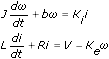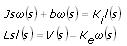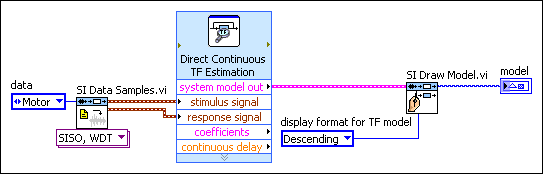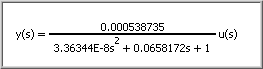# Identifying Continuous-Time Transfer Function Models (System Identification Toolkit)

LabVIEW 2013 System Identification Toolkit Help

Edition Date: June 2013

Part Number: 372458D-01

»View Product InfoDownload Help (Windows Only)

After physical modeling, you can describe the direct circuit (DC) servomotor by using the following differential equations:If you want to design an analog proportional-integral-derivative (PID) controller, perform the Laplace transform on these differential equations to obtain the following equations:Set Ki to be equal to Ke to transform these differential equations. You can transform these differential equations to the following continuous-time transfer function model:where K is the electromotive force constant. ω is the rotational speed of the shaft. U is the driving voltage. J is the moment of inertia of the DC servomotor. b is the damping ratio of the mechanical part of the DC servomotor. R is the electric resistance. L is the inductance of circuit.

In this example, you need to estimate the values of K, JL, (bL+JR), and (bR+K2) to identify the continuous-time transfer function model.

## Using the SI Transfer Function Estimation Express VI

Complete the following steps to identify the continuous-time transfer function model by using the SI Transfer Function Estimation Express VI.

1. Add the SI Transfer Function Estimation Express VI to the block diagram of the VI you created.

The Configure SI Transfer Function Estimation dialog box appears.
2. Select the Waveform button to identify dynamic models by using waveform input signals.
3. Click the Model Settings tab.
4. Select the Continuous button to identify continuous-time transfer function models.
5. Enter 0 in the Numerator order text box because the numerator of this model is constant only.
6. Enter 2 in the Denominator order text box because the highest denominator order of the continuous-time transfer function model is s2.
7. Click the OK button to close the configuration dialog box and return to the block diagram.
8. Wire the stimulus signal and response signal outputs of the SI Data Samples VI to the corresponding inputs of the SI Transfer Function Estimation Express VI.
9. Add the SI Draw Model VI to the block diagram.

10. Wire the system model out output of the SI Transfer Function Estimation Express VI to the system model input of the SI Draw Model VI.
11. (Optional) Create a constant for the display format for TF model input of the SI Draw Model VI and set the value to Descending.
12. Create an indicator for the model output of the SI Draw Model VI. The following figure shows the block diagram.13. Wire all error in and error out terminals on the block diagram.
14. Run this VI to identify the dynamic system model.

## Using the SI Estimate Transfer Function Model VI

Complete the following steps to identify the continuous-time transfer function model by using the SI Estimate Transfer Function Model VI.

1. Add the SI Estimate Transfer Function Model VI to the block diagram of the VI you created.

2. Wire the stimulus signal and response signal outputs of the SI Data Samples VI to the corresponding inputs of the SI Estimate Transfer Function Model VI.
3. Create a control for the orders of transfer function model input of the SI Estimate Transfer Function Model VI.
4. Enter 0 in the num order field of this control because the numerator of this model is constant only.
5. Enter 2 in the den order field of this control because the highest denominator order of this model is s2.
6. (Optional) Switch to the front panel, right-click this control, and select Data Operations»Make Current Value Default from the shortcut menu. LabVIEW uses the current value as the default value for this control.
7. Add the SI Draw Model VI to the block diagram.

8. Wire the system model out output of the SI Estimate Transfer Function Model VI to the system model input of the SI Draw Model VI.
9. (Optional) Create a constant for the display format for TF model input of the SI Draw Model VI and set the value to Descending.
10. Create an indicator for the model output of the SI Draw Model VI. The following figure shows the block diagram.11. Wire all error in and error out terminals on the block diagram.
12. Run this VI to identify the dynamic system model.

## Verifying Resulting Dynamic System Models

If you use the SI Data Samples VI to generate the input and output data in Part 2, LabVIEW returns the following system model:where K=0.000538735. JL=3.36326E–8. (bL+JR)=0.0658172. (bR+K2)=1.